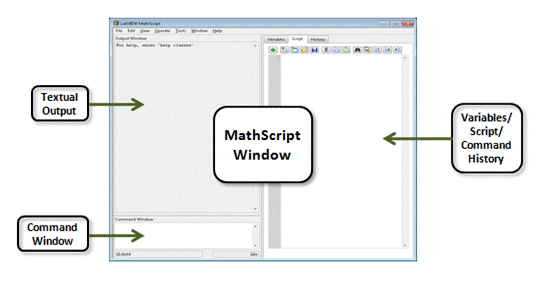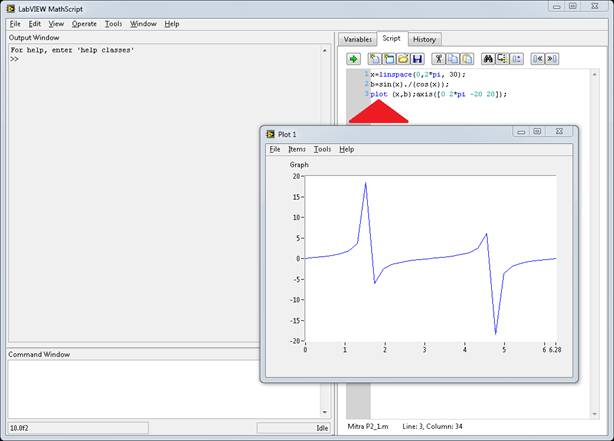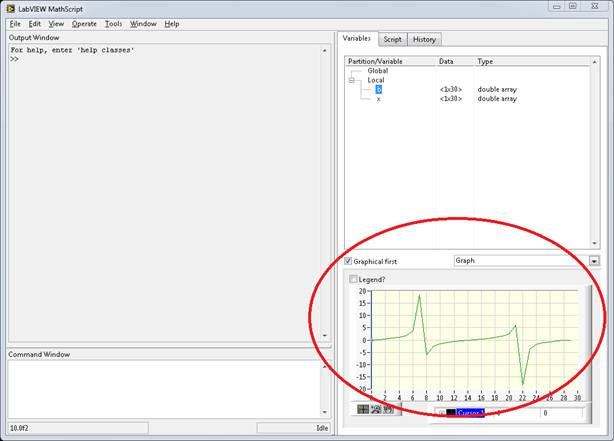# Developing Algorithms Using LabVIEW MathScript RT Module: Part 2- The LabVIEW MathScript Window

Publish Date: Nov 28, 2018 | 20 Ratings | 3.80 out of 5 | Print | 1 Customer Review | Submit your review

## Overview

This tutorial provides detailed information on working with the MathScript Interactive Window as part of the LabVIEW MathScript RT Module.

### 1. What Is the LabVIEW MathScript RT Module?

LabVIEW MathScript RT is an add-on module for the LabVIEW Full and Professional Development Systems. It is designed to natively add text-based signal processing, analysis, and math into the graphical development environment of LabVIEW. With more than 800 built-in functions, LabVIEW MathScript gives you the ability to either run your existing custom .m files or create them from scratch. Using this native solution for text-based math, you can combine graphical and textual programming within LabVIEW because the text-based engine is part of the LabVIEW environment. With LabVIEW MathScript RT, you can choose whether graphical or textual programming is most appropriate for each aspect of your application.

### 2. How Do I Use the MathScript RT Module?

There are two methodologies for interacting with the MathScript Engine: the MathScript Interactive Window and the MathScript Node.

### Using the MathScript Node

The MathScript Node, accessed from the LabVIEW block diagram functions palette, provides an interface to MathScript that gives you the power to combine graphical and textual programming within the same LabVIEW application. By passing variables across the border of the structure, the MathScript Node is designed to help you deploy your scripts.

For more detailed information on using the MathScript Node, read “Part 1 – The MathScript Node.”

### Using the MathScript Interactive Window

The MathScript Interactive Window, accessed from the Tools menu, provides an intuitive interface to MathScript. With a command-line interface and a window to build batch files, the MathScript Interactive Window is designed to help you develop your scripts.

You can use the MathScript Interactive Window to enter commands one at time. You also can enter batch scripts in a simple text editor window, loaded from a text file, or imported from a separate text editor. The MathScript Interactive Window provides immediate feedback in a variety of forms, such as graphs and text.Figure 1. Use the MathScript Interactive Window for algorithm development.

1. In the getting started window, select Tools » MathScript Window to open the MathScript Interactive Window.
2. In the command window, enter 4+6+1. Then press the <Enter> key. LabVIEW displays the result of the command in the output window. You can enter commands one at a time for immediate feedback.
3. Click the Variables tab. LabVIEW updates the variable, ans, under the Local section in the Partition/Variable tree, to contain the result of the last command executed.
4. Click the Script tab and enter the following commands:

x=linspace(0,2*pi, 30);
b=sin(x)./(cos(x));
plot (x,b);axis([0 2*pi -20 20]);

Notice that the x=linspace(0,2*pi,30); command creates a new variable x and populates that variable with 30 samples evenly distributed between 0 and 2*pi.
5. Click the Run button on the Script page. The plot 1 window appears and displays an XY plot of x versus b.Figure 2. The MathScript Interactive Window provides a standard
“command-line” interface typical of .m file environments.

6. Click the Variables tab to display variables you create, such as x and b.
7. Select b from the Partition/Variable tree. A table representing the numeric values of b appears. Select the Graphical First? checkbox to change the numeric display to a graph display. A graph of the results appears. When you select the Graphical First? checkbox, you see the graphical display of the variable’s values first, rather than the default of the numerical representation.Figure 3. Use the Variables tab in the MathScript Interactive Window to visualize your script variables.

8. Click the Script tab and click the Load button. Select Mitra P2_1.m (Mitra, Sanjit and Kaiser, James H., Handbook for Digital Signal Processing [New York: John Wiley and Sons, 1993], 21). This script generates a test signal and then applies a moving-average filter to the test signal.
9. Click the Run button to run the script. The Prompt User for Input dialog box appears. Enter a positive value in the Desired length of the filter = text box and click the OK button. MathScript scripts can include interactive dialog boxes to request user input.
10. The Mitra P2_1.m script, shown below, uses subplot commands to specify one of the four subplots on the plot window. After you specify a subplot, subsequent commands affect that subplot. For example, a plot command, following a subplot command, populates the data specified in the subplot command with the data specified in the plot command.

% Program P2_1
% Simulation of an M-point Moving Average Filter
% Generate the input signal
n = 0:100;
s1 = cos(2*pi*0.05*n); % A low-frequency sinusoid
s2 = cos(2*pi*0.47*n); % A high-frequency sinusoid
x = s1+s2;
% Implementation of the moving-average filter
M = input('Desired length of the filter = ');
num = ones(1,M);
y = filter(num,1,x)/M;
% Display the input and output signals
clf;
subplot(2,2,1);
plot(n, s1);
axis([0, 100, -2, 2]);
xlabel('Time index n'); ylabel('Amplitude');
title('Signal #1');
subplot(2,2,2);
plot(n, s2);
axis([0, 100, -2, 2]);
xlabel('Time index n'); ylabel('Amplitude');
title('Signal #2');
subplot(2,2,3);
plot(n, x);
axis([0, 100, -2, 2]);
xlabel('Time index n'); ylabel('Amplitude');
title('Input Signal');
subplot(2,2,4);
plot(n, y);
axis([0, 100, -2, 2]);
xlabel('Time index n'); ylabel('Amplitude');
title('Output Signal');
axis;

11. You can use the command window to find more information about commands. For example, type help subplot in the window and a description of the command, along with information about syntax, inputs, outputs, and examples, appears.
Customer Reviews
1 Review | Submit your review

- Mar 4, 2008

Superb example clearly its bridging the usual DSP scripts mostly done in MatLab that now doable in a LabVIEW environment - it would be good if a hardware or IO from a script could be demonstrated also